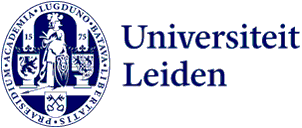#Research programme

# Algebra, Geometry and Number Theory

The research of the Algebra, Geometry and Number Theory programme ranges from fundamental mathematical theory to algorithms and applications.

Contact
Peter Bruin

The researchers in this programme work on diverse topics in fundamental mathematics. This research covers areas such as number theory, cryptology and algebraic geometry. There is a strong emphasis on effective methods and computational aspects. Our research helps answering important questions within mathematics. For example, we develop methods for solving equations when the solutions have to be whole numbers, and study what happens when higher-dimensional geometric objects are deformed. Our work also has applications to the development of mathematical methods for cryptography, which is used in securing digital data and internet communication.